# The Importance Of Cross Validation In Machine Learning

Dear all,

This article gives some background in layman terms on the importance of cross validation in machine learning. It includes the K-fold cross validation that Jim @Jrinne is currently doing outside P123.

Regards
James

# The Importance Of Cross Validation In Machine Learning

Before we analyze the importance of cross validation in machine learning, let us look at the definition of cross validation.

## What is Cross Validation?

Cross validation is a technique for assessing how the statistical analysis generalizes to an independent dataset.

Before testing out any model, would you not like to test it with an independent dataset? Normally, in any prediction problem, your model works on a known dataset. You also call it the training dataset. However, in real-time, your model will have to work on an unknown dataset.

Under such circumstances, will your model be able to predict the outcome correctly? You do not know unless you test your model on a random dataset. This testing is what we refer to as cross validation. Once your model passes this test, it is fit to work anywhere.

### The Purpose of Cross Validation

The purpose of cross validation is to assess how your prediction model performs with an unknown dataset. We shall look at it from a layman’s point of view.

You are learning how to drive a car. Now, anyone can drive a car on an empty road. The real test is how you drive in demanding traffic. It is why the trainers train you on roads that have traffic so that you get used to it.

Therefore, when it is time for you actually to drive your car, you are prepared to do so without the trainer sitting by your side to guide you. You are ready to handle any situation, the like of which you might not have encountered before.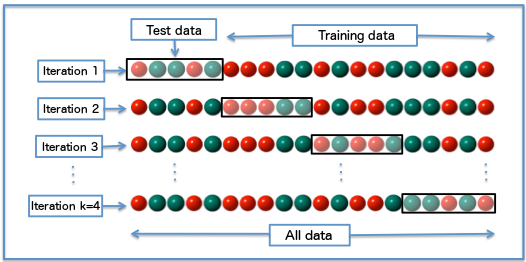Prediction model

### Different Types of Cross Validation in Machine Learning

There are two types of cross validation:

(A) Exhaustive Cross Validation – This method involves testing the machine on all possible ways by dividing the original sample into training and validation sets.

(B) Non-Exhaustive Cross Validation – Here, you do not split the original sample into all the possible permutations and combinations.

#### (A) Exhaustive Cross Validation

There are two types of exhaustive cross validation in machine learning

1. Leave-p-out Cross Validation (LpO CV)

Here you have a set of observations of which you select a random number, say ‘p.’ Treat the ‘p’ observations as your validating set and the remaining as your training sets.

There is a disadvantage because the cross validation process can become a lengthy one. It depends on the number of observations in the original sample and your chosen value of ‘p.’

2. Leave-one-out Cross Validation (LOOCV)

This method of cross validation is similar to the LpO CV except for the fact that ‘p’ = 1. The advantage is that you save on the time factor.

However, if the number of observations in the original sample is large, it can still take a lot of time. Nevertheless, it is quicker than the LpO CV method.

#### (B) Non-Exhaustive Cross validation

As the name suggests, you do not compute all the ways of splitting the original sample. Hence, you can also call it the approximations of the LpO CV.

1. K-fold Cross Validation

This method envisages partitioning of the original sample into ‘k’ equal sized sub-samples. You take out a single sample from these ‘k’ samples and use it as the validation data for testing your model. You treat the remaining ‘k-1’ samples as your training data.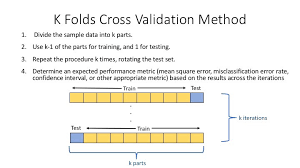K folds cross validation method

You repeat the cross validation process ‘k’ times using each ‘k’ sample as the validation data once. Take an average of the ‘k’ number of results to produce your estimation. The advantage of this method is that you use all the observations for both training and validation, and each sample for validation once.

2. Stratified K-fold Cross Validation

This procedure is a variation of the method described above. The difference is that you select the folds in such a way that you have equal mean response value in all the folds.

3. Holdout Method

The holdout cross validation method is the simplest of all. In this method, you randomly assign data points to two sets. The size of the sets does not matter.

Treat the smaller set say ‘d0’ as the testing set and the larger one, ‘d1’ as the training set. You train your model on the d0 set and test it on the d1. There is a disadvantage because you do a single run. It can give misleading results.

4. Monte Carlo Cross Validation

This test is a better version of the holdout test. You split the datasets randomly into training data and validation data. For each split, you assess the predictive accuracy using the respective training and validation data.

Finally, you average the results over all the splits. The advantage of this method is that the proportion of the validation or training split is not dependent on the number of folds (K-fold test). However, there is a disadvantage as well.

There are chances that you might miss out some observations whereas you might select some observations more than once. Under such circumstances, the validation subsets could overlap. You also refer to this procedure as Repeated Random Sub-sampling method.

### The Importance of Cross Validation in Machine Learning

When your original validation partition does not represent the overall population, you get a model that might appear to have a high degree of accuracy.

However, in reality, it will not be much use because it can work with a particular set of data. The moment it finds data outside its purview, the machine cannot recognize it thereby resulting in poor accuracy.

When you use cross validation in machine learning, you verify how accurate your model is on multiple and different subsets of data. Therefore, you ensure that it generalizes well to the data that you collect in the future. It improves the accuracy of the model.

### Limitations of Cross Validation

We have seen what cross validation in machine learning is and understood the importance of the concept. It is a vital aspect of machine learning, but it has its limitations.

(a) In an ideal world, the cross validation will yield meaningful and accurate results. However, the world is not perfect. You never know what kind of data the model might encounter in the future.

(b) Usually, in predictive modelling, the structure you study evolves over a period. Hence, you can experience differences between the training and validation sets. Let us consider you have a model that predicts stock values.

You have trained the model using data of the previous five years. Would it be realistic to expect accurate predictions over the next five-year period?

(c) Here is another example where the limitation of the cross validation process comes to the fore. You develop a model for predicting the individual’s risk of suffering from a particular ailment.

However, you train the model using data from a study involving a specific section of the population. The moment you apply the model to the general population, the results could vary a lot.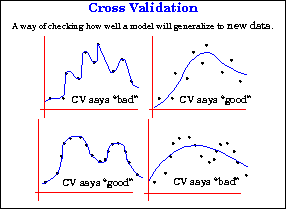Cross validation – a way of checking

### Applications of Cross Validation

(a) You can use cross validation to compare the performances of a set of predictive modelling procedures.

(b) It has excellent use in the field of medical research. Consider that we use the expression levels of a certain number of proteins, say 15 for predicting whether a cancer patient will respond to a specific drug.

The ideal way is to determine which subset of the 15 features produce the ideal predictive model. Using cross validation, you can determine the exact subset that provides the best results.

(c) Recently, data analysts have used cross validation in the field of medical statistics. These procedures are useful in the meta-analysis.

### Cross validation in Python

Cross validation has applications in Python. It is useful for preventing overfitting and underfitting. Let us now see the applications of cross validation in Python.

Overfitting occurs when you train the model “too well.” It happens when you have a complex model with numerous variables as compared to the number of observations.

Under such circumstances, the model will prove to be great while on the training mode but might not be accurate on new data. It is because it is not a generalized model.

As opposed to overfitting, underfitting occurs when the model does not fit the training data. Hence, you cannot generalize it to new data.

It is because you are looking at a basic model that does not have enough independent variables. It can also happen when you fit a linear model to a non-linear data.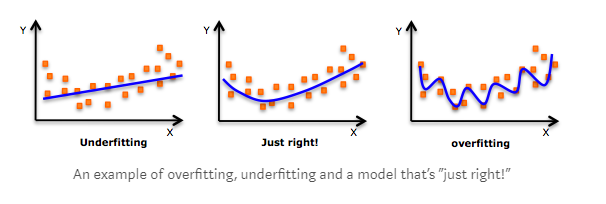Both overfitting and underfitting are undesirable in data analysis. You should always aim for a balanced approach or a model that is ‘just right.’ Cross-validation can help you prevent overfitting and underfitting.

You can use the K-Fold Cross validation and the LOOCV processes to solve these issues. We have already seen how these processes work. Here is a visual description of the K-Fold CV method.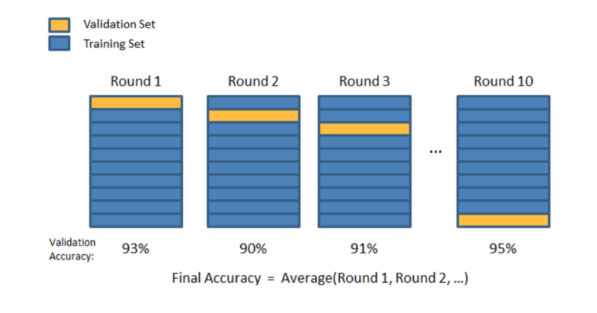K-fold cv method

You have ten varying results. Take out the average of the ten results to get the final accuracy figure.

### Wrap Up

Machine learning requires a lot of analysis of data. Cross validation is an ideal method to prepare the machine face real-time situations.

Consequently, the machine becomes ready to assimilate new data and generalize it to deliver accurate predictions.